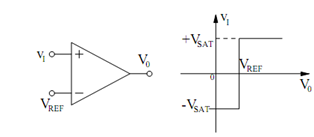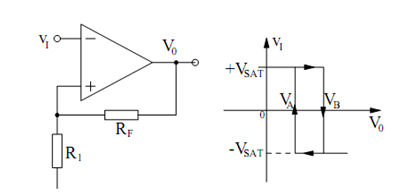## Non-inverting Comparator Assignment Help

Assignment Help: >> Sinusoidal Oscillator Using Op-amps - Non-inverting Comparator

Non-inverting Comparator:

Figure (a) illustrates the circuit diagram of an op-amp utilized as a non-inverting comparator. The output voltage of the circuit is given by

v0  = VSAT sgn (VI - VREF )

The graph of v0 versus vI  is given in Figure(a)                                            (b)

Figure: (a) Non-inverting Comparator; and (b) Plot of v0 Versus vI.

Positive feedback is frequently utilized with comparator circuits. The feedback is applied through the output to the non-inverting input of the op-amp. It is in contrast to the circuits covered in the preceding sections where feedback is applied to the inverting input. (The non-inverting integrator is an exception that uses feedback to both op-amp inputs.) Figure (a) gives the circuit diagram of an inverting op-amp comparator with positive feedback. The circuit is also called as a Schmidt trigger. The capacitor CF in the figure is supposed to be an open circuit in the following. This capacitor is frequently used to develop the switching speed of a comparator by enhancing the amount of positive feedback at high frequencies. It has no effect on the input voltage at which the op-amp switches states.(a)                                                  (b)

Figure: (a) Inverting Comparator with Positive Feedback; and (b) Plot of v0 Versus vI.

The output voltage from the circuit of Figure (a) may be written

v0  = VSAT sgn (V+  - VI )

Because v0 has two stable states, v0 = +VSAT and v0 = - VSAT, it follows that vI may have two stable states given by

VA   = V REF ( RF / (RF +R1)  ) - VSAT (R1 /RF  + R1)

VB   = V REF ( RF / (RF +R1)  ) - VSAT (R1 /RF  + R1)

where superposition and voltage division have been used for each equation. For

vI  < VA, it follows that v0 = +VSAT. For vI > VB, it follows that v0 = - VSAT. For VA < vI < VB, v0 may have two stable states, i.e. v0 = ± VSAT. The graph of v0 versus vI is given in Figure (b).

The value of v0 for VA < vI   < VB based on whether vI   enhance from a value less than VA or vI reduce from a value greater than VB. that is, the circuit has memory. If vI  < VA, primarily and vI starts to increase, v0 remains at the + VSAT   state until vI   becomes greater than VB. At this point, v0 switches to the - VSAT   state. If vI > VB primarily and vI starts to decrease, v0  remains at the - VSAT   state until vI becomes less than VA. Then v0 switches to the + VSAT state. The path for v0 on the graph in Figure (b) is mentioned with arrows. The loop in the graph is commonly called a hysteresis loop.